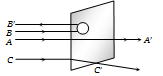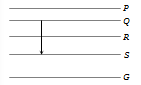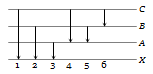A beam of fast moving alpha particles were directed towards a thin film of gold. The parts A',B', and C' of the transmitted and reflected beams corresponding to the incident parts A, B and C of the beam, are shown in the adjoining diagram. The number of alpha particles in(a) B' will be minimum and in C' maximum
(b) A' will be maximum and in B' minimum
(c) A' will be minimum and in B' maximum
(d) C' will be minimum and in B' maximum

Concept Questions :-

Various atomic model
High Yielding Test Series + Question Bank - NEET 2020

Difficulty Level:

According to Bohr's theory the radius of electron in an orbit described by principal quantum number n and atomic number Z is proportional to
(a) ${\mathrm{Z}}^{2}{\mathrm{n}}^{2}$            (b) $\frac{{\mathrm{Z}}^{2}}{{\mathrm{n}}^{2}}$
(c) $\frac{{\mathrm{Z}}^{2}}{\mathrm{n}}$              (d) $\frac{{\mathrm{n}}^{2}}{\mathrm{Z}}$

Concept Questions :-

Bohr's model of atom
High Yielding Test Series + Question Bank - NEET 2020

Difficulty Level:

The radius of electron's second stationary orbit in Bohr's atom is R. The radius of the third orbit will be

(a) 3 R                    (b) 2.25 R
(c) 9 R                    (d) $\frac{\mathrm{R}}{3}$

Concept Questions :-

Bohr's model of atom
High Yielding Test Series + Question Bank - NEET 2020

Difficulty Level:

If m is mass of electron, v its velocity, r the radius of stationary circular orbit around a nucleus with charge Ze, then from Bohr's first postulate, the kinetic energy $\mathrm{K}=\frac{1}{2}{\mathrm{mv}}^{2}$ of the electron in C.G.S. system is equal to

(a) $\frac{1}{2}\frac{{\mathrm{Ze}}^{2}}{\mathrm{r}}$                             (b) $\frac{1}{2}\frac{{\mathrm{Ze}}^{2}}{{\mathrm{r}}^{2}}$
(c) $\frac{{\mathrm{Ze}}^{2}}{\mathrm{r}}$                                 (d) $\frac{{\mathrm{Ze}}^{2}}{{\mathrm{r}}^{2}}$

Concept Questions :-

Bohr's model of atom
High Yielding Test Series + Question Bank - NEET 2020

Difficulty Level:

In any Bohr orbit of the hydrogen atom, the ratio of kinetic energy to potential energy of the electron is
(a) 1/2                    (b) 2
(c) $-1/2$                (d) – 2

Concept Questions :-

Bohr's model of atom
High Yielding Test Series + Question Bank - NEET 2020

Difficulty Level:

The spectral series of the hydrogen spectrum that lies in the ultraviolet region is the

(a) Balmer series                 (b) Pfund series
(c) Paschen series                (d) Lyman series

Concept Questions :-

Spectral series
High Yielding Test Series + Question Bank - NEET 2020

Difficulty Level:

Figure shows the energy levels P, Q, R, S and G of an atom where G is the ground state. A red line in the emission spectrum of the atom can be obtained by an energy level change from Q to S. A blue line can be obtained by following energy level change(a) P to Q (b) Q to R
(c) R to S (d) R to G

Concept Questions :-

Spectral series
High Yielding Test Series + Question Bank - NEET 2020

Difficulty Level:

A hydrogen atom (ionisation potential 13.6 eV) makes a transition from third excited state to first excited state. The energy of the photon emitted in the process is
(a) 1.89 eV                (b) 2.55 eV
(c) 12.09 eV              (d) 12.75 eV

Concept Questions :-

Bohr's model of atom
High Yielding Test Series + Question Bank - NEET 2020

Difficulty Level:

The figure indicates the energy level diagram of an atom and the origin of six spectral lines in emission (e.g. line no. 5 arises from the transition from level B to A). The following spectral lines will also occur in the absorption spectrum(a) 1, 4, 6                      (b) 4, 5, 6
(c) 1, 2, 3                      (d) 1, 2, 3, 4, 5, 6

Concept Questions :-

Spectral series
High Yielding Test Series + Question Bank - NEET 2020

Difficulty Level:

When a hydrogen atom is raised from the ground state to an excited state

(a) P.E. increases and K.E. decreases

(b) P.E. decreases and K.E. increases

(c) Both kinetic energy and potential energy increase

(d) Both K.E. and P.E. decrease

Concept Questions :-

Various atomic model